# 6-Speed Lepelletier

Clutch schedule for a six-speed Lepelletier transmission

• Library:
• Simscape / Driveline / Transmissions

•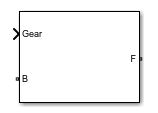## Description

The 6-Speed Lepelletier consists of one planetary gear set, one Ravigneaux gear set, and five disk friction clutches. The transmission base shaft connects to the ring gear of the planetary gear set. The follower shaft connects to the ring gear of the Ravigneaux gear set. Three of the clutches control the power flow paths between the planetary and Ravigneaux gear sets. The other two clutches serve as brakes, grounding various gears of the Ravigneaux set to the transmission housing.

This diagram shows a six-speed Lepelletier transmission. The labels for the gear components are superimposed on the input and output gears. The table lists the gear and clutch components that are labeled in the diagram.LabelComponent
P.G.Planetary gear
R.G.Ravigneaux gear
RRing gear
CPlanet gear carrier
SSun gear
SLLarge sun gear
SSSmall sun gear
A–CForward clutches that control the power flow path
D–EForward, braking clutches

### Drive Ratios, Clutch Schedule, and Power Flow

The drive ratio between the transmission input and output shafts follows from the elementary gear ratios specified for the gear blocks. The elementary gear ratios are

`${g}_{1}=\frac{{N}_{PR}}{{N}_{PS}},$`

`${g}_{2}=\frac{{N}_{RR}}{{N}_{RSL}},$`

and

`${g}_{3}=\frac{{N}_{RR}}{{N}_{RSS}},$`

where:

• NPR is the number of teeth in the planetary ring gear.

• NPS is the number of teeth in the planetary sun gear.

• NRR is the number of teeth in the Ravigneaux ring gear.

• NRSL is the number of teeth in the Ravigneaux large sun gear.

• NRRS is the number of teeth in the Ravigneaux small sun gear.

The table shows the clutch schedule, drive-ratio expressions, drive-ratio default values, and the power-flow diagrams for each gear of the 6-Speed Lepelletier block.

The letters in the clutch schedule columns denote the brakes and clutches. A value of `1` denotes a locked state and a value of `0` an unlocked state. The clutch schedule generates these signals based on the Gear port input signal. The signals are scaled through a Gain block and used as actuation inputs in the clutch blocks.

The power-flow diagrams show the power flow paths between input and output shafts for each gear setting. Power flow is shown in orange. Connections to the transmission housing (a mechanical ground) are shown in black.

GearClutch ScheduleDrive Ratio EquationDefault RatioPower Flow
ABCDE
601001$\frac{{g}_{2}}{1+{g}_{2}}$0.69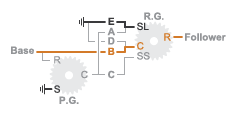511000$\frac{{g}_{2}}{{g}_{2}+1/\left(1+{g}_{1}\right)}$0.87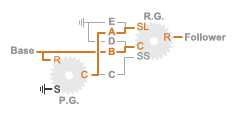401100$\frac{{g}_{3}\left(1+{g}_{1}\right)}{{g}_{3}\left(1+{g}_{1}\right)-1}$1.14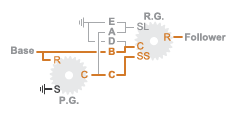310100$\frac{1+{g}_{1}}{{g}_{1}}$1.50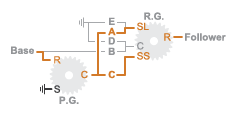200101$\frac{\left(1+{g}_{1}\right)\left({g}_{3}+{g}_{2}\right)}{{g}_{1}\left(1+{g}_{2}\right)}$2.31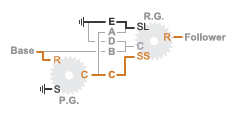100110$\frac{{g}_{3}\left(1+{g}_{1}\right)}{{g}_{1}}$4.12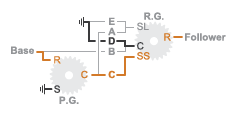R10010$-\frac{{g}_{2}\left(1+{g}_{1}\right)}{{g}_{1}}$-3.36## Ports

### Input

expand all

Specify -1 for reverse, 0 for neutral, and 1–6 for the forward gears.

Data Types: `single` | `double`

### Conserving

expand all

Conserving port associated with the base shaft.

Conserving port associated with the follower shaft.

## Parameters

expand all

Sets the initial engagement or disengagement of the clutches using the clutch schedule. When the transmission is in neutral, there is no power flow from the base shaft to the follower shaft.

## Version History

Introduced in R2015a